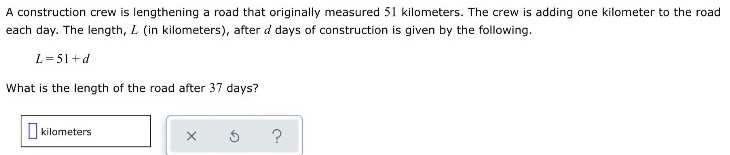### Still have math questions?

Algebra
QuestionA construction crew is lengthening a road that originally measured $$5$$ I kilometers. The crew is adding one kilometer to the road each day. The length, $$L$$ (in kilometers), after $$d$$ days of construction is given by the following.

$$L = 51 + d$$

What is the length of the road after $$37$$ days?